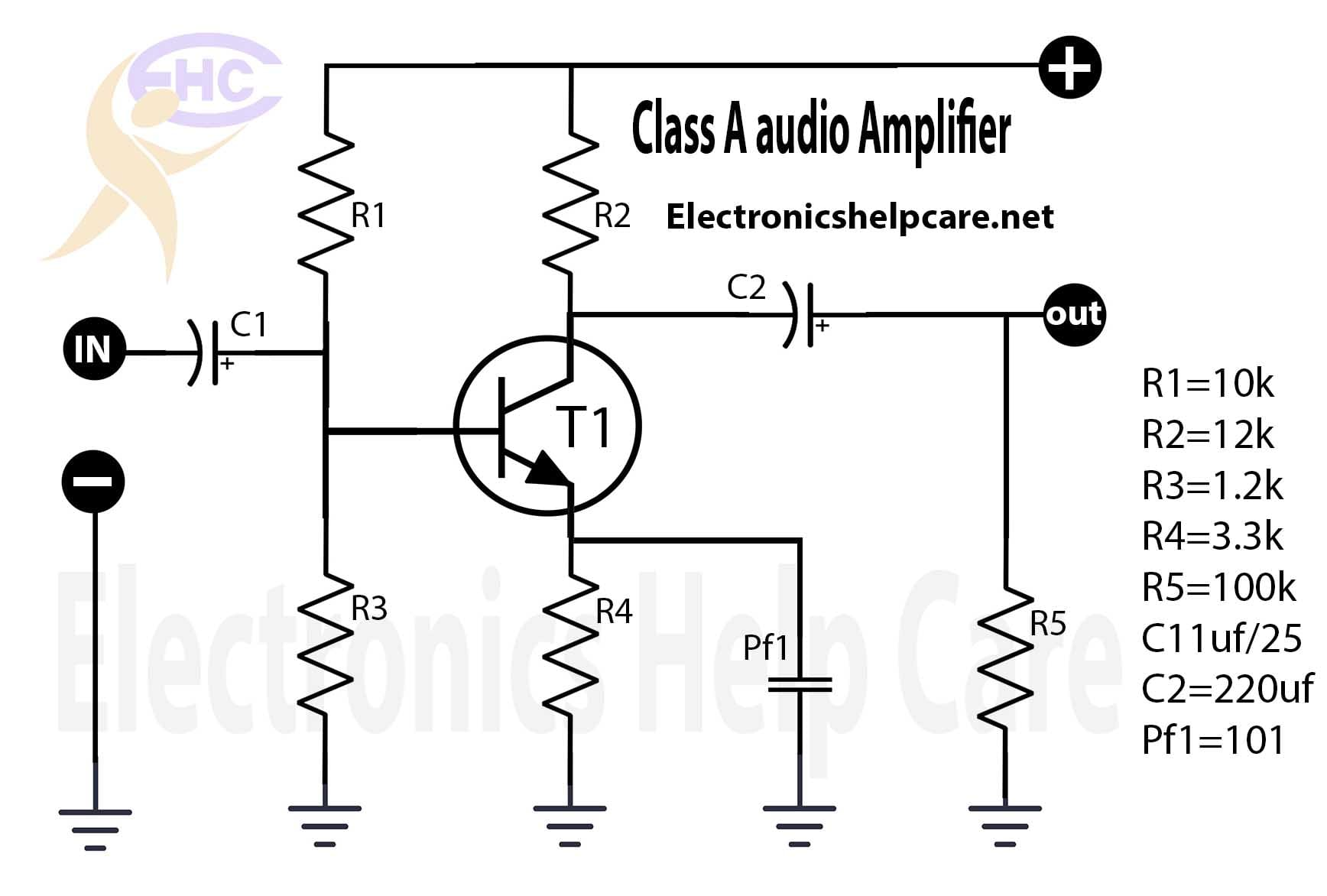Breaking News

# Class a amplifier circuit

This is the Class A amplifier circuit diagram. we can use 12 voltage. negative and positive voltage. it’s a mono audio diagram. this is a simple and easy diagram. we also can use this as a pre-amplifier. this is the logic of making an amplifier.For this circuit, we need 12 voltage 2 amperes transformer. all resistors will be 1/4 watts. R1 is 10k. R2=12k. R3=1.2k. R4=3.3k. R5=100k. C1=1uf/25volt. C2=220uf/25volt. Pf1=101.

transistor diagram of 2sa1943 and 2sc5200

Build a Transformer or construct the transformer. Home Theater Circuit Diagram 5.1

Thanks a lot for being with us. If you want another post then please visit our website.

We have another post for you. like repairing

or  Diagram